# High School Math : Perpendicular Lines

## Example Questions

2 Next →

### Example Question #4 : How To Find The Slope Of A Perpendicular Line

Which of the following is perpendicular to the line described by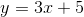Possible Answers: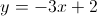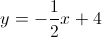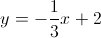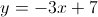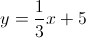Correct answer:Explanation:

The definition of perpendicular lines is that their slopes are inverse reciprocals of one another. Since the slope in the given equation is, this means that the slope of its perpendicular line would be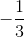.

The answer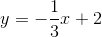is the only equation listed that has a slope of.

### Example Question #5 : How To Find The Slope Of A Perpendicular Line

Which of the following is perpendicular to the line described by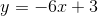Possible Answers: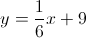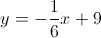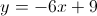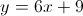Correct answer:Explanation:

The definition of perpendicular lines is that their slopes are inverse reciprocals of one another. Since the slope in the given equation is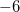, this means that the slope of its perpendicular line would be.

The answer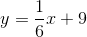is the only equation listed that has a slope of.

### Example Question #6 : How To Find The Slope Of A Perpendicular Line

Which of the following gives the slope of a line that is perpendicular to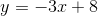?

Possible Answers:Correct answer:Explanation:

Recall that the slopes of perpendicular lines are opposite reciprocals of one another. As a result, we are looking for the opposite reciprocal of. Thus, we can get that the opposite reciprocal is### Example Question #7 : How To Find The Slope Of A Perpendicular Line

Find the slope of the line perpendicular to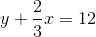.

Possible Answers: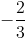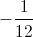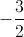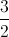Correct answer:Explanation:

Put this equation into slope-intercept form, y = mx + b, to find the slope, m.

Do this by subtracting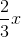from both sides of the equation: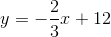The slope of this line is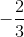.

The slope of the perpendicular line is the negative reciprocal. Switch the numerator and denominator, and then multiply by: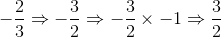### Example Question #8 : How To Find The Slope Of A Perpendicular Line

What is the slope of the line perpendicular to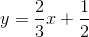?

Possible Answers: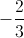Correct answer:Explanation:

In standardform,is the slope.

The slope of a perpendicular line is the negative reciprocal of the original line.

For our given line, the slope is. Therefore, the slope of the perpendicular line is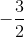.

### Example Question #9 : How To Find The Slope Of A Perpendicular Line

Find the slope of this line: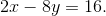Possible Answers: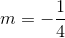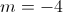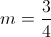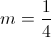Correct answer:Explanation:

Isolate forso that the equation now reads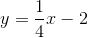The slope is### Example Question #41 : Coordinate Geometry

Which of the following are perpendicular to the line with the formula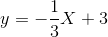?

I.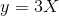II.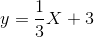III.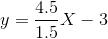Possible Answers:

I and III

I

II and III

II

III

Correct answer:

I and III

Explanation:

The slope of a perpendicular line is equal to the negative reciprocal of the original line.  This means that the slope of our perpendicular line must be 3.  We can also note that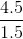is also equal to 3, so both of these slopes are correct.  The y-intercept does not matter, as the slope is the only thing that determines the slant of the line.  Therefore, numerals I and III are both correct.

### Example Question #11 : Perpendicular Lines

Which of the following lines is perpendicular to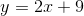?

Possible Answers: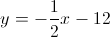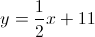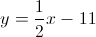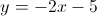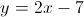Correct answer:Explanation:

In order for two lines to be perpendicular to each other, their slopes must be opposites and reciprocals of each other, meaning the fraction must be flipped upside down and the signs must be changed. In this situation, the original equation had a slope of, so the perpendicular slope must be### Example Question #43 : Coordinate Geometry

Which of the following lines will be perpendicular to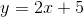?

Possible Answers: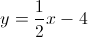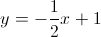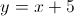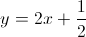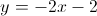Correct answer:Explanation:

Two lines are perpendicular if they have opposite reciprocal slopes. When a line is in standardform, theis the slope. A perpendicular line will have a slope of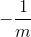.

The slope of our given line is. Therefore we want a slope of. The only line with the correct slope is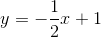.

2 Next →

### All High School Math Resources